# 字节面试必须拿下的十道算法题，你会几道？

#### 前言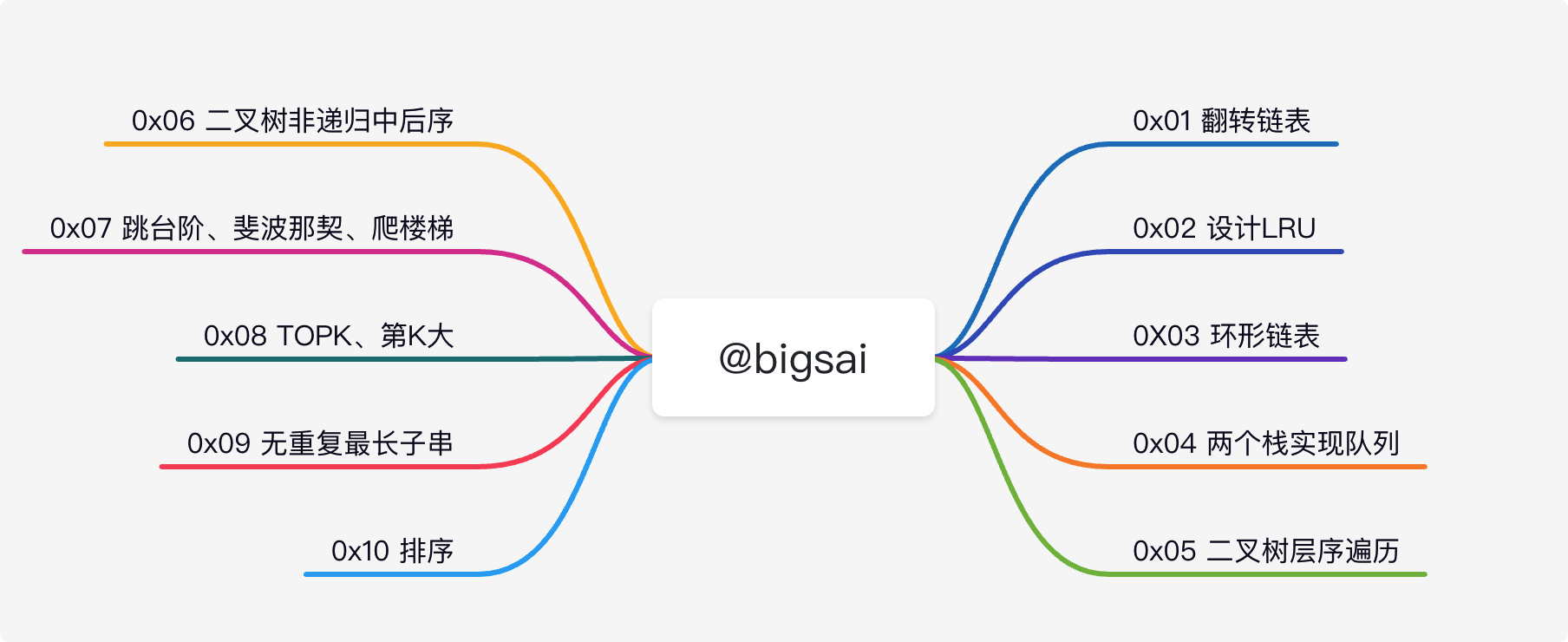#### 0X01翻转链表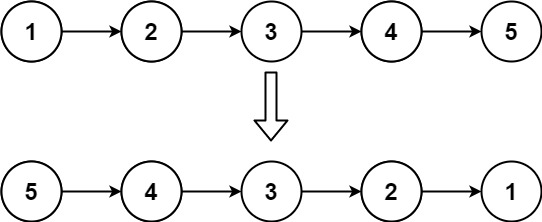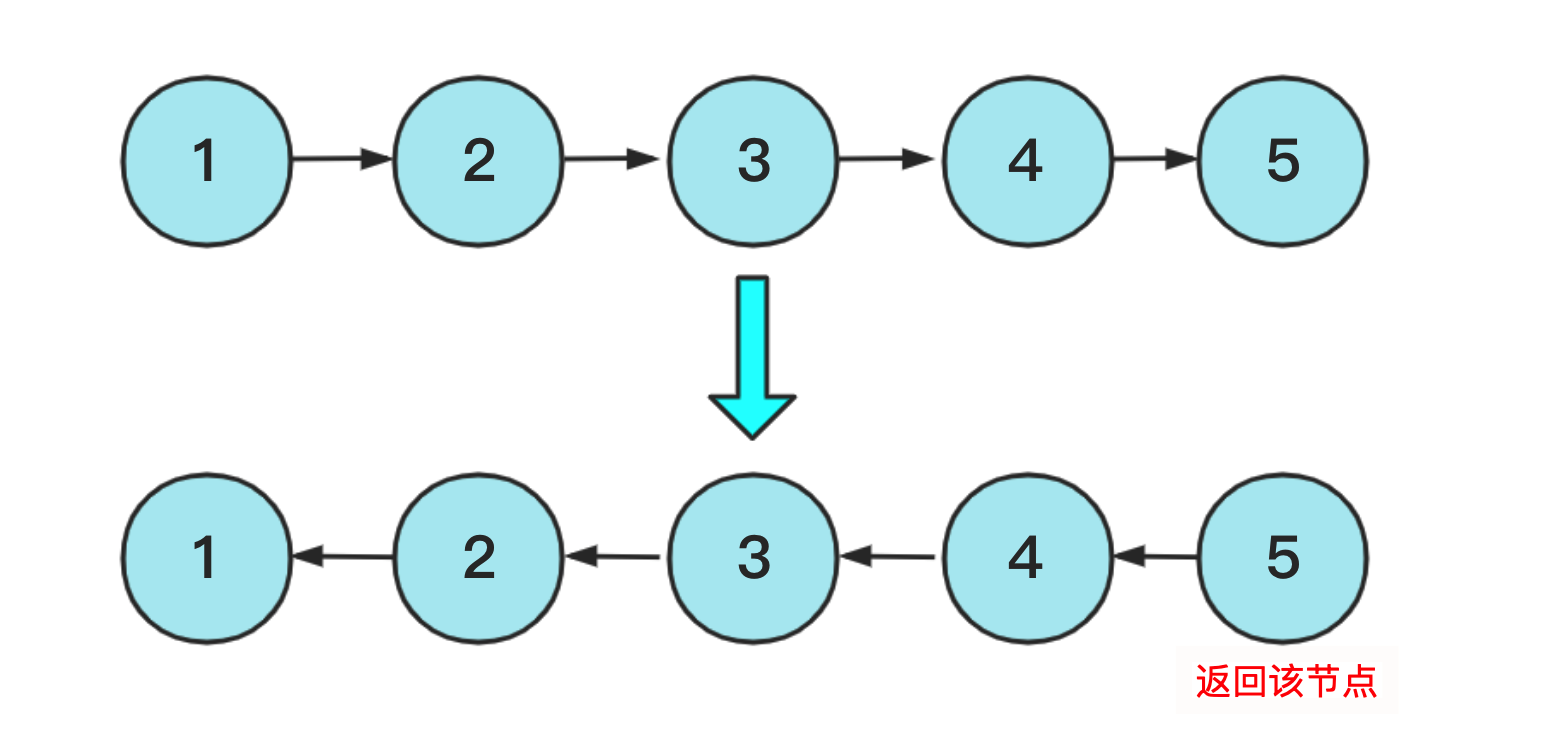class Solution {
while (cur!=null)
{
ListNode next=cur.next;
//改变指向
cur.next=pre;
pre=cur;
cur=next;
}
return pre;
}
}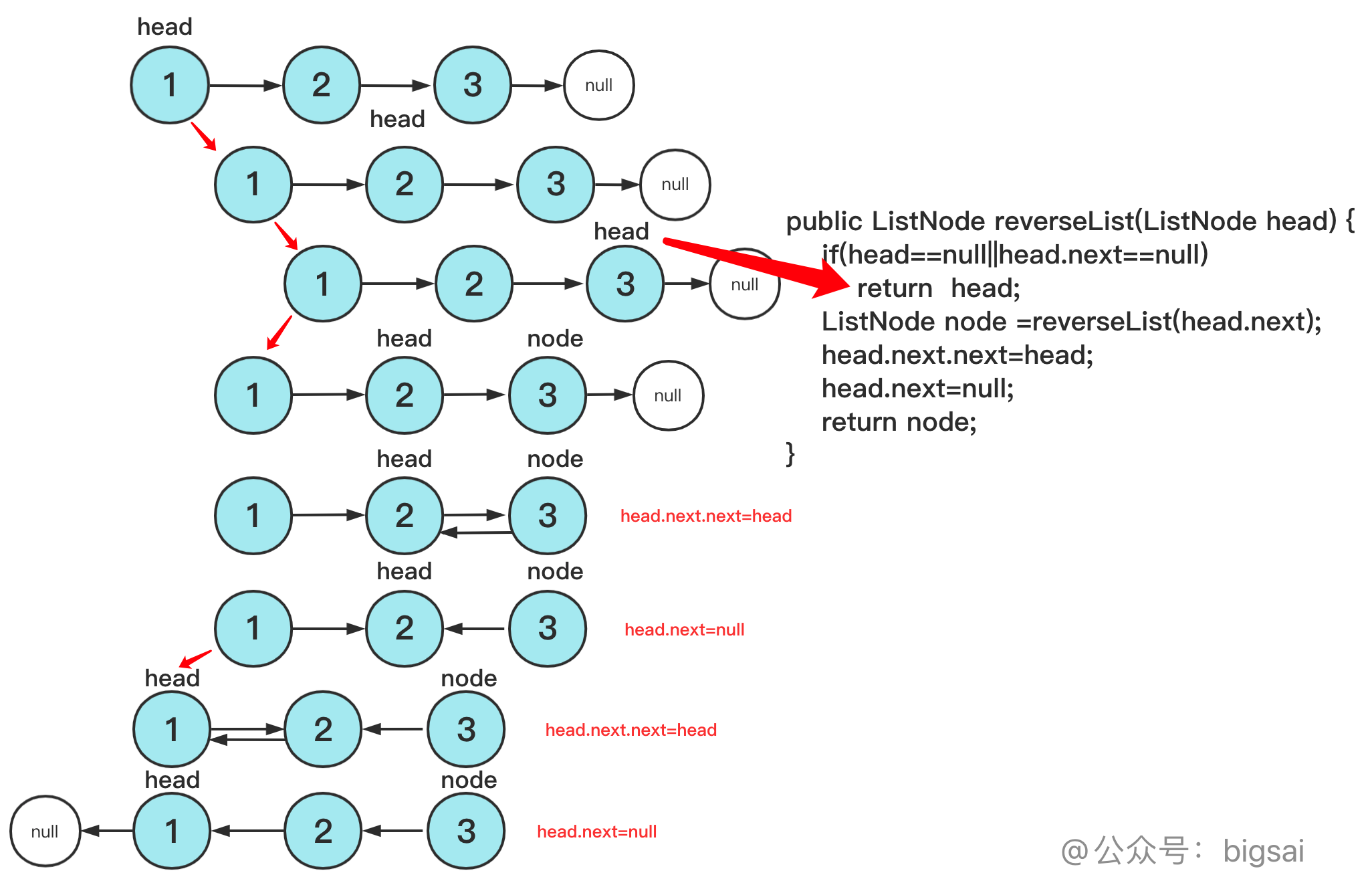class Solution {
return node;//返回最后一个节点(一直被递归传递)
}
}


#### 0X02设计LRU

LRUCache(int capacity) 以正整数作为容量 capacity 初始化 LRU 缓存
int get(int key) 如果关键字 key 存在于缓存中，则返回关键字的值，否则返回 -1 。
void put(int key, int value) 如果关键字已经存在，则变更其数据值；如果关键字不存在，则插入该组「关键字-值」。当缓存容量达到上限时，它应该在写入新数据之前删除最久未使用的数据值，从而为新的数据值留出空间。

LRU的核心就是借助哈希+双链表，哈希用于查询，双链表实现删除只知道当前节点也能O(1)的复杂度删除，不过双链表需要考虑的头尾指针特殊情况。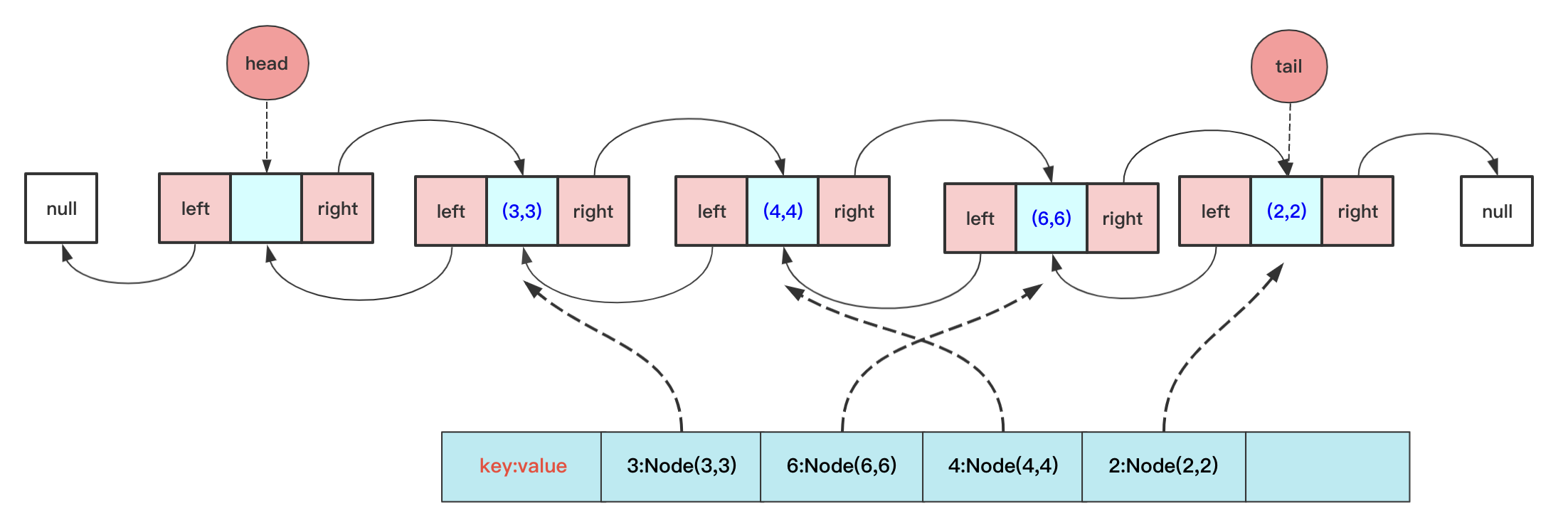class LRUCache {
class Node {
int key;
int value;
Node pre;
Node next;
public Node() {
}
public Node( int key,int value) {
this.key = key;
this.value=value;
}
}
class DoubleList{
private Node tail;// 尾节点
private int length;
public DoubleList() {
length = 0;
}
{
tail.next = teamNode;
teamNode.pre=tail;
tail = teamNode;
length++;
}
void deleteFirst(){
return;

length--;
}
void deleteNode(Node team){

team.pre.next=team.next;
if(team.next!=null)
team.next.pre=team.pre;
if(team==tail)
tail=tail.pre;
team.pre=null;
team.next=null;
length--;
}
}
Map<Integer,Node> map=new HashMap<>();
DoubleList doubleList;//存储顺序
int maxSize;

public   LRUCache(int capacity) {
doubleList=new DoubleList();
maxSize=capacity;
}
public int get(int key) {
int val;
if(!map.containsKey(key))
return  -1;
val=map.get(key).value;
Node team=map.get(key);
doubleList.deleteNode(team);
return  val;
}

public void put(int key, int value) {
if(map.containsKey(key)){// 已经有这个key 不考虑长短直接删除然后更新
Node deleteNode=map.get(key);
doubleList.deleteNode(deleteNode);
}
else if(doubleList.length==maxSize){//不包含并且长度小于
map.remove(first.key);
doubleList.deleteFirst();
}
Node node=new Node(key,value);
map.put(key,node);

}
}


#### 0X03环形链表

public class Solution {
ListNode slow=fast;
while (fast!=null&&fast.next!=null) {
slow=slow.next;
fast=fast.next.next;
if(fast==slow)
return true;
}
return false;
}
}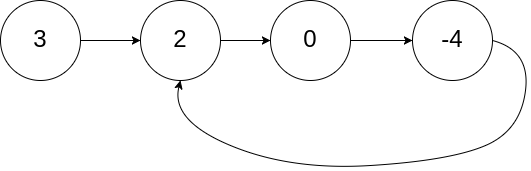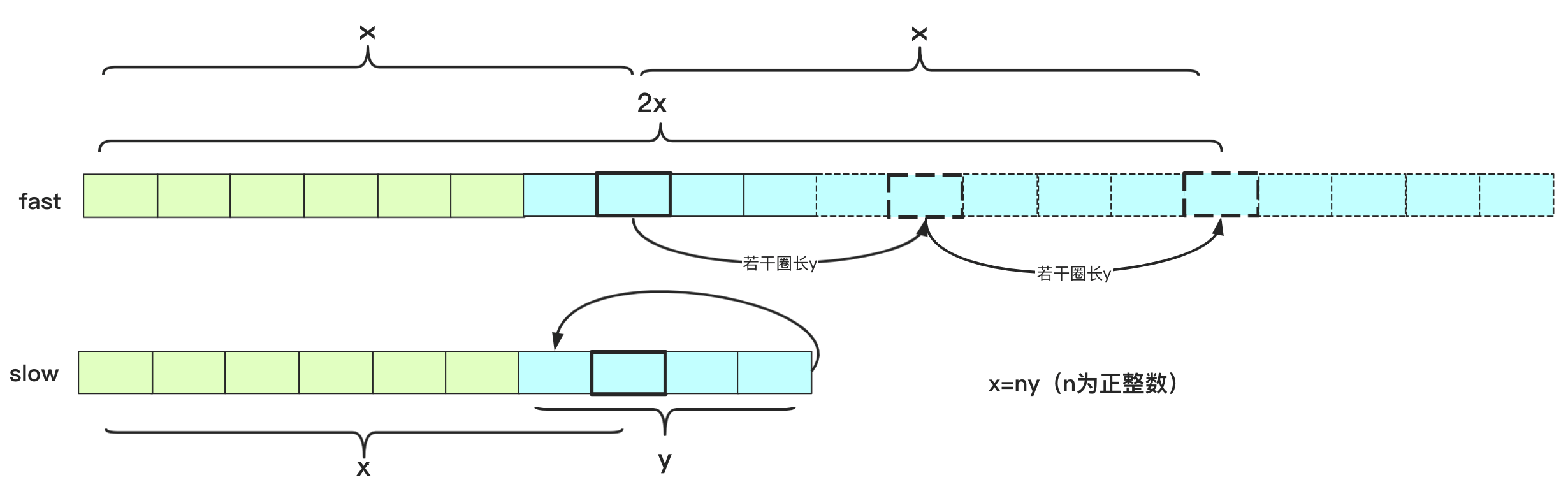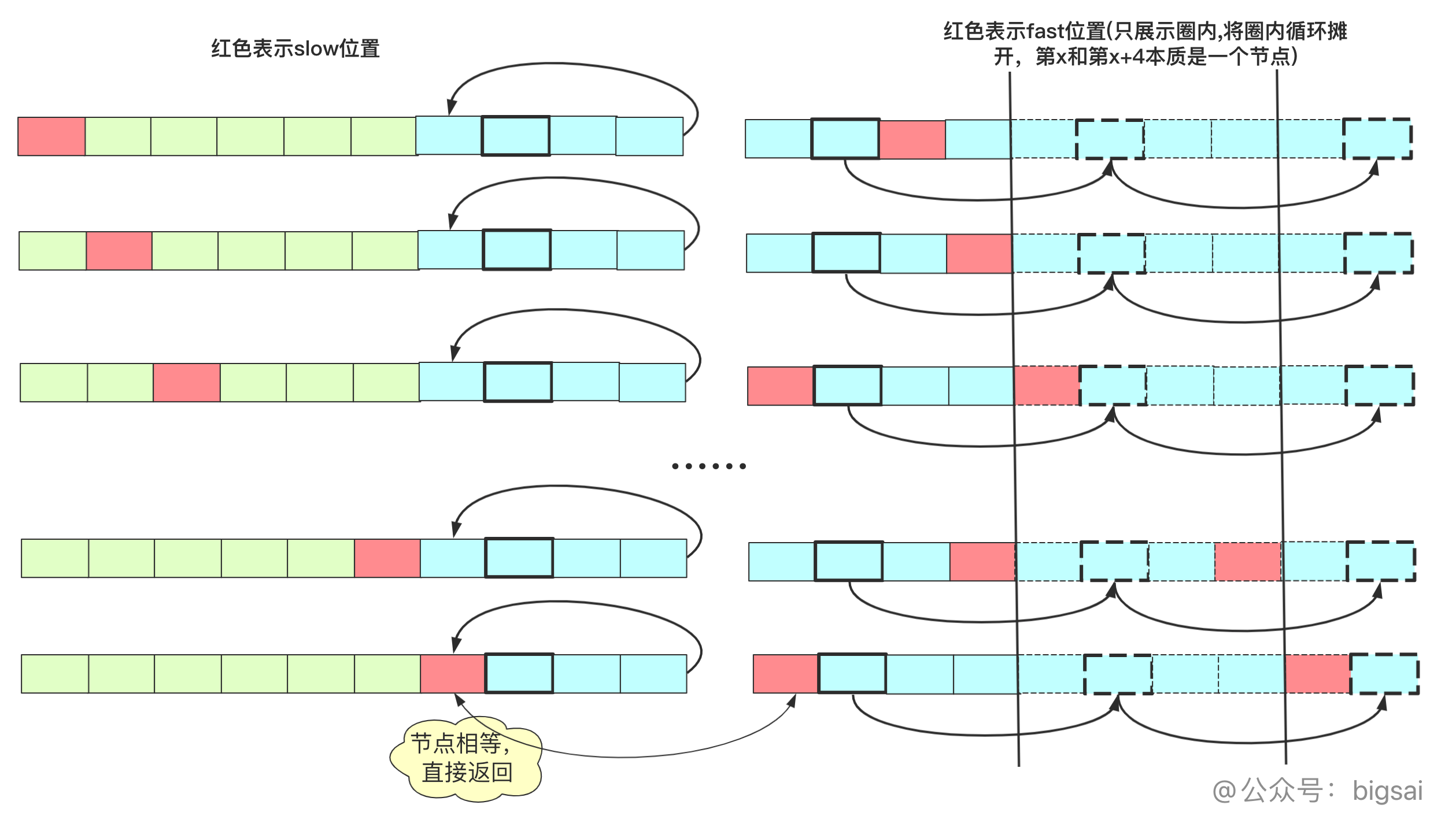public class Solution {
boolean isloop=false;
ListNode fast=new ListNode(0);//头指针
ListNode slow=fast;
if(fast.next==null||fast.next.next==null)
return null;
while (fast!=null&&fast.next!=null) {
fast=fast.next.next;
slow=slow.next;
if(fast==slow)
{
isloop=true;
break;
}
}
if(!isloop)//如果没有环返回
return null;
while (team!=fast) {//slow 和fast 分别从起点和当前点出发
team=team.next;
fast=fast.next;
}
return team;
}
}


#### 0X04两个栈实现队列

class CQueue {

Stack<Integer>stack1=new Stack<>();
Stack<Integer>stack2=new Stack<>();
public CQueue() {
}
public void appendTail(int value) {
stack1.push(value);
}
if(stack1.isEmpty())
return -1;

while (!stack1.isEmpty())
{
stack2.push(stack1.pop());
}
int value= stack2.pop();
while (!stack2.isEmpty())
{
stack1.push(stack2.pop());
}
return  value;
}
}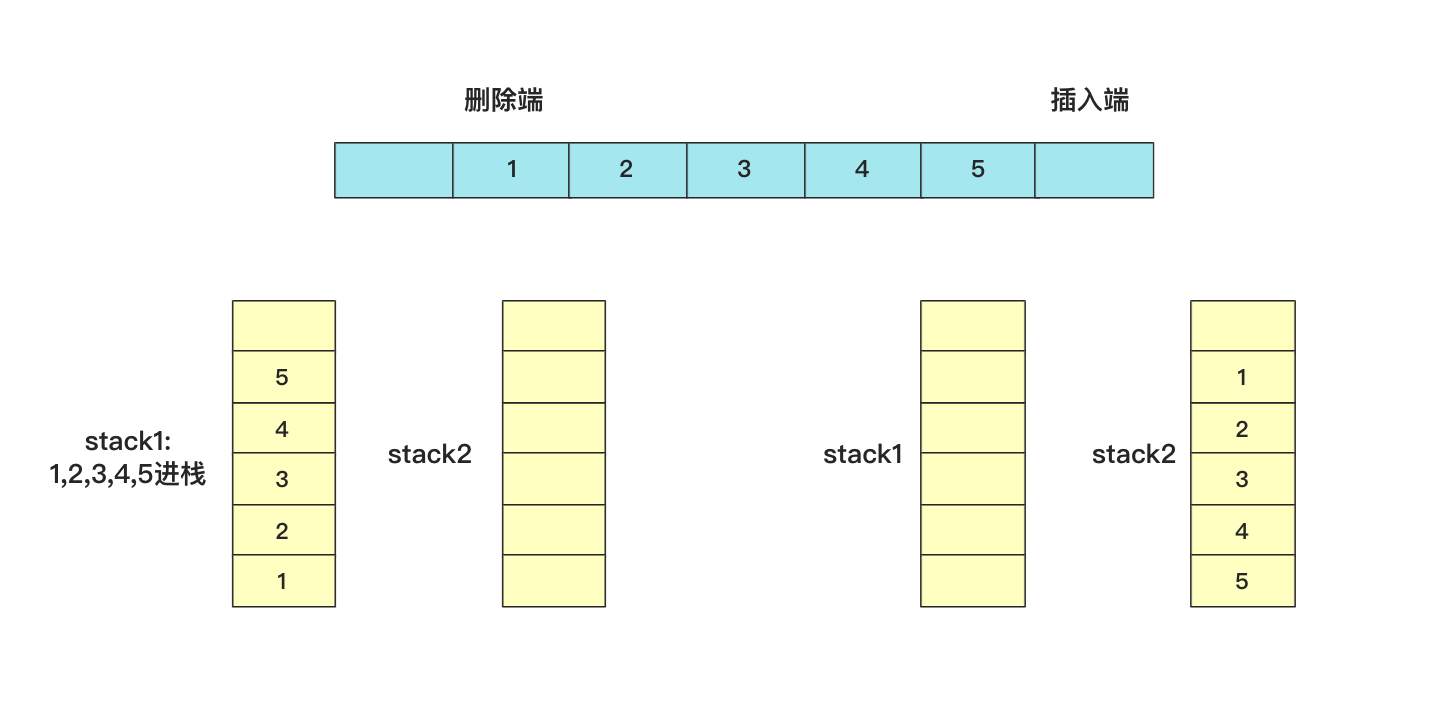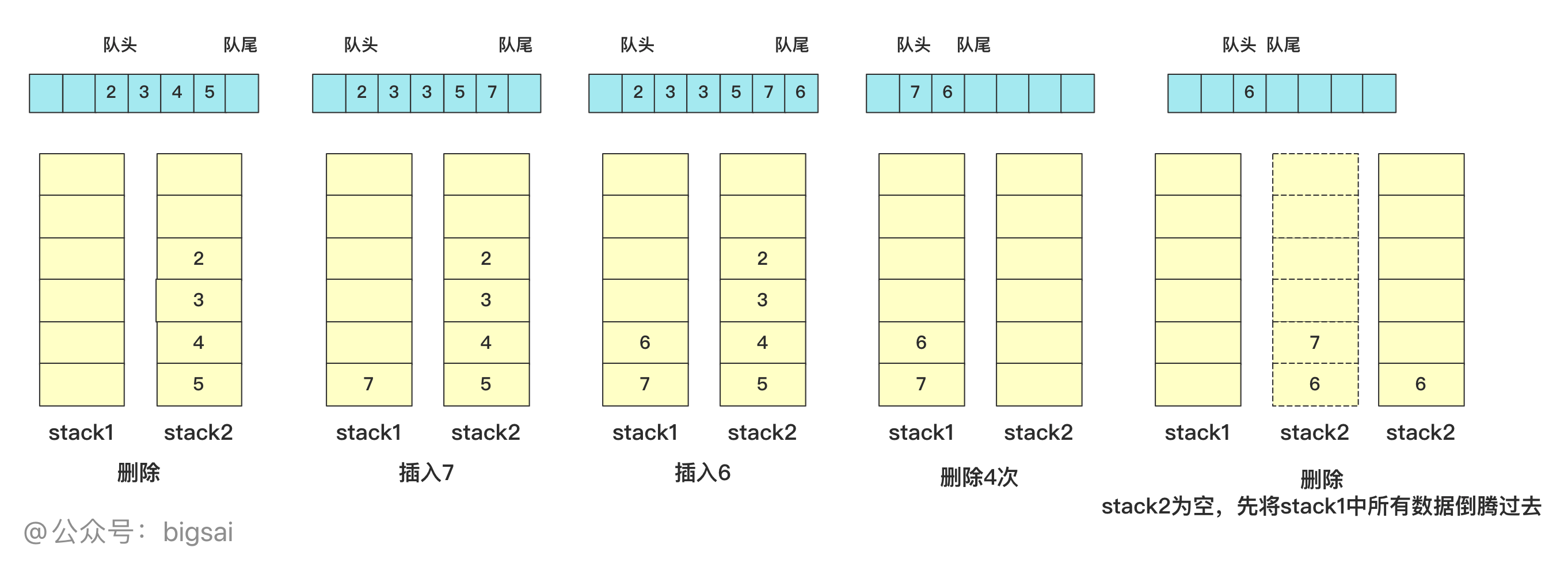class CQueue {
Deque<Integer> stack1;
Deque<Integer> stack2;

public CQueue() {
}

public void appendTail(int value) {
stack1.push(value);
}

// 如果第二个栈为空 将stack1数据加入stack2
if (stack2.isEmpty()) {
while (!stack1.isEmpty()) {
stack2.push(stack1.pop());
}
} //如果stack2依然为空 说明没有数据
if (stack2.isEmpty()) {
return -1;
} else {//否则删除
int deleteItem = stack2.pop();
return deleteItem;
}
}
}


#### 0X05二叉树层序(锯齿)遍历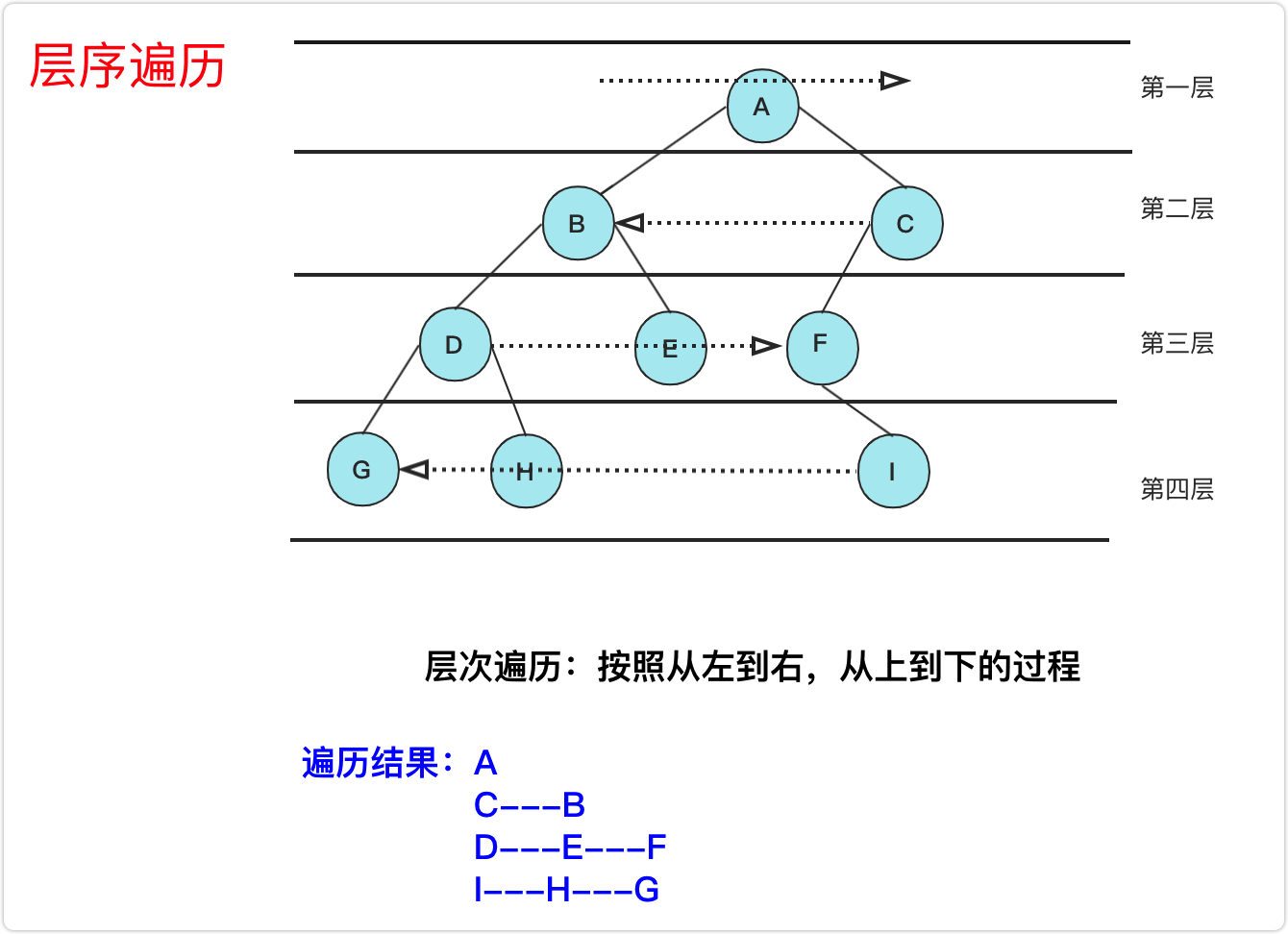public List<List<Integer>> levelOrder(TreeNode root) {
List<List<Integer>> value=new ArrayList<>();//存储到的最终结果
if(root==null)
return value;
int index=0;//判断
Queue<TreeNode>queue=new ArrayDeque<>();
while (!queue.isEmpty()){
List<Integer>va=new ArrayList<>();//临时 用于存储到value中
int len=queue.size();//当前层节点的数量
for(int i=0;i<len;i++){
TreeNode node=queue.poll();
if(index%2==0)//根据奇偶 选择添加策略
else
if(node.left!=null)
if(node.right!=null)
}
index++;
}
return value;
}


#### 0X06 二叉树中后序遍历(非递归)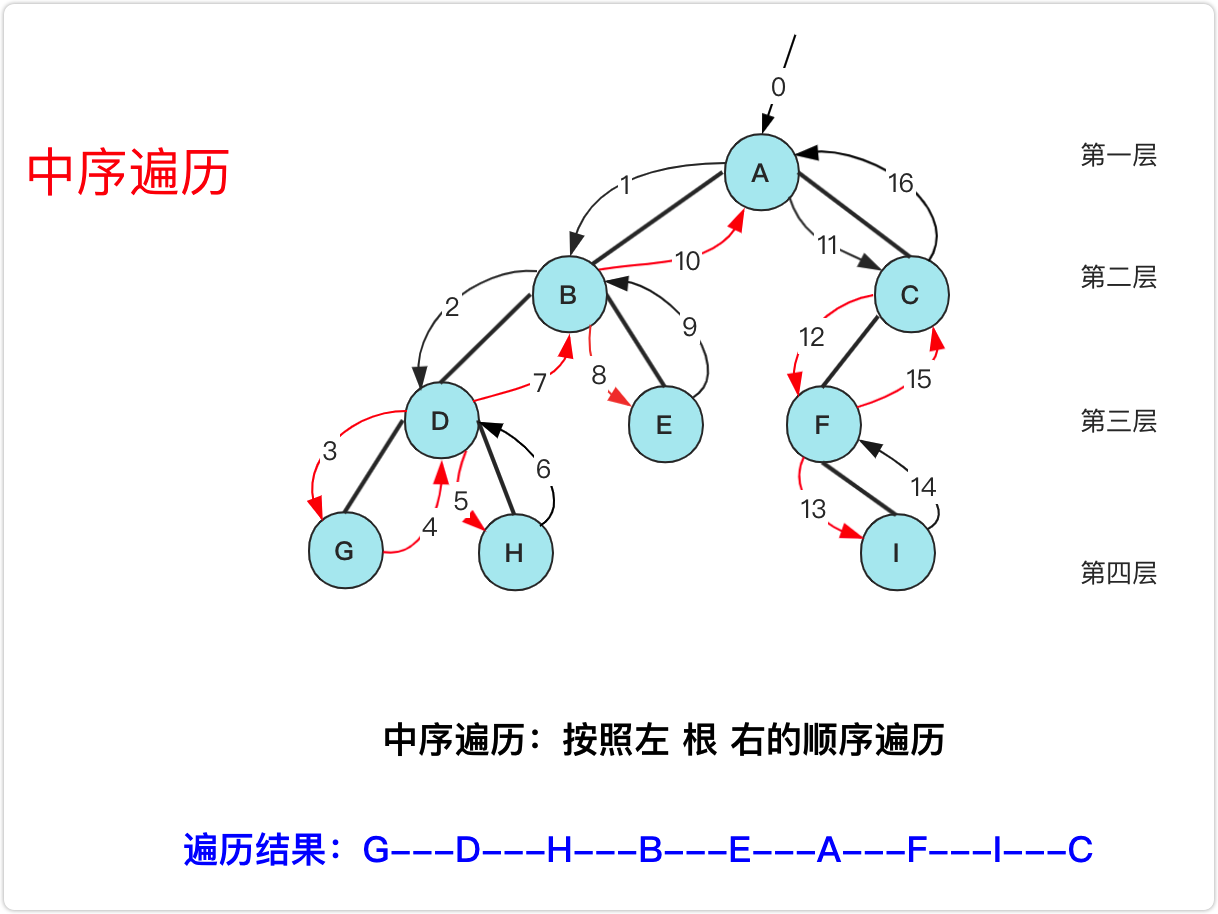1. 枚举当前节点(不存储输出)并用栈存储，节点指向左节点，直到左孩子为null。
2. 抛出栈顶访问。如果有右节点，访问其右节点重复步骤1，如有没右节点，继续重复步骤2抛出。

class Solution {
public List<Integer> inorderTraversal(TreeNode root) {
List<Integer>value=new ArrayList<Integer>();
Stack<TreeNode> q1 = new Stack();
while(!q1.isEmpty()||root!=null)
{
while (root!=null) {
q1.push(root);
root=root.left;
}
root=q1.pop();//抛出
root=root.right;//准备访问其右节点

}
return value;
}
}


class Solution {
public List<Integer> postorderTraversal(TreeNode root) {
TreeNode temp=root;//枚举的临时节点
List<Integer>value=new ArrayList<>();
TreeNode pre=null;//前置节点
Stack<TreeNode>stack=new Stack<>();

while (!stack.isEmpty()||temp!=null){

while(temp!=null){
stack.push(temp);
temp=temp.left;
}
temp=stack.pop();
if(temp.right==pre||temp.right==null)//需要弹出
{
pre=temp;
temp=null;//需要重新从栈中抛出
}else{
stack.push(temp);
temp=temp.right;
}

}
return value;
}
}


#### 0X07 跳台阶(斐波那契、爬楼梯)

class Solution {
public int climbStairs(int n) {
if(n<3)return n;
int dp[]=new int[n+1];
dp=1;
dp=2;
for(int i=3;i<n+1;i++)
{
dp[i]=dp[i-1]+dp[i-2];
}
return dp[n];
}

public int climbStairs(int n) {
int a = 0, b = 0, c = 1;
for (int i = 1; i <= n; i++) {
a = b;
b = c;
c = a + b;
}
return c;
}
}


#### 0X08 TOPK问题

TOPK问题真的非常经典，通常问的有最小的K个数，寻找第K大都是TOPK这种问题，这里就用力扣215寻找数组第K大元素作为板子。

TOPK的问题解决思路有很多，如果优化的冒泡或者简单选择排序，时间复杂度为O(nk),使用优化的堆排序为O(n+klogn)，不过掌握快排的变形就可以应付大体上的所有问题了(面试官要是让你手写堆排序那真是有点难为你了)。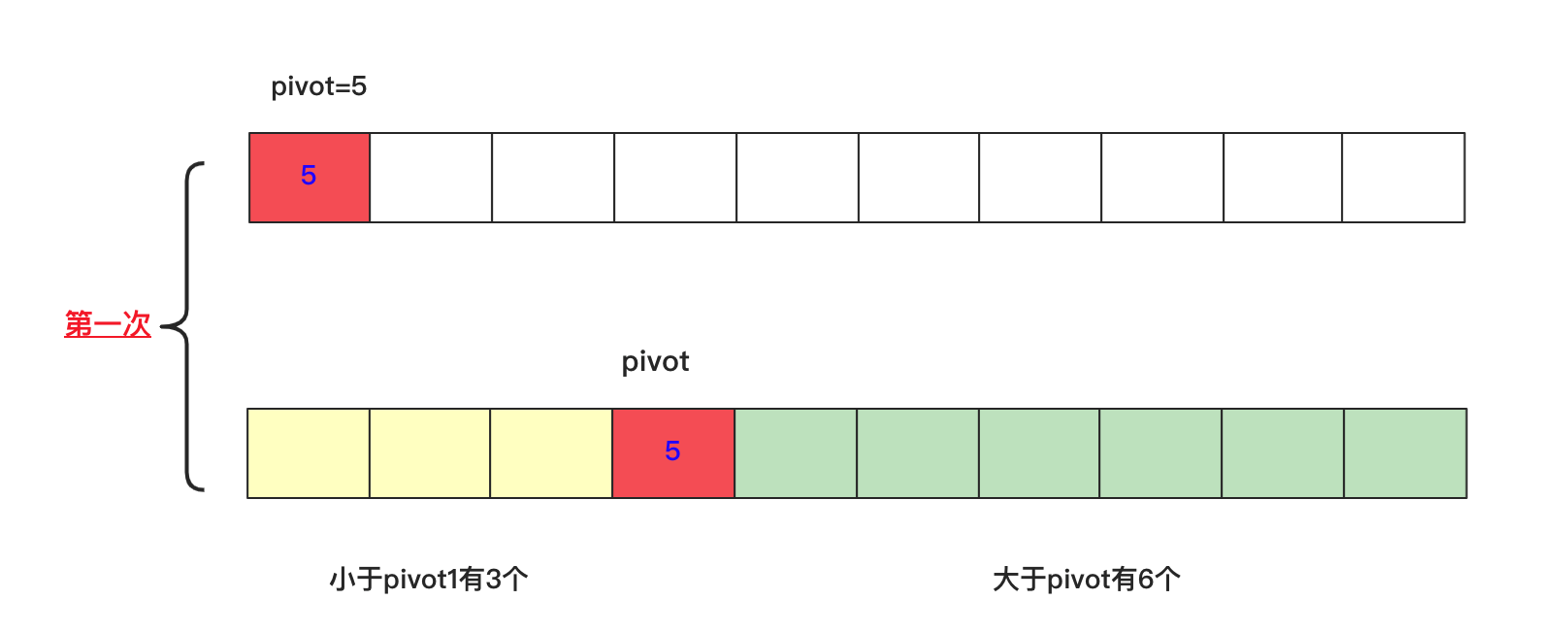class Solution {
public int findKthLargest(int[] nums, int k) {
quickSort(nums,0,nums.length-1,k);
return nums[nums.length-k];
}
private void quickSort(int[] nums,int start,int end,int k) {
if(start>end)
return;
int left=start;
int right=end;
int number=nums[start];
while (left<right){
while (number<=nums[right]&&left<right){
right--;
}
nums[left]=nums[right];
while (number>=nums[left]&&left<right){
left++;
}
nums[right]=nums[left];
}
nums[left]=number;
int num=end-left+1;
if(num==k)//找到k就终止
return;
if(num>k){
quickSort(nums,left+1,end,k);
}else {
quickSort(nums,start,left-1,k-num);
}
}
}


#### 0X09 无重复的最长子串(数组)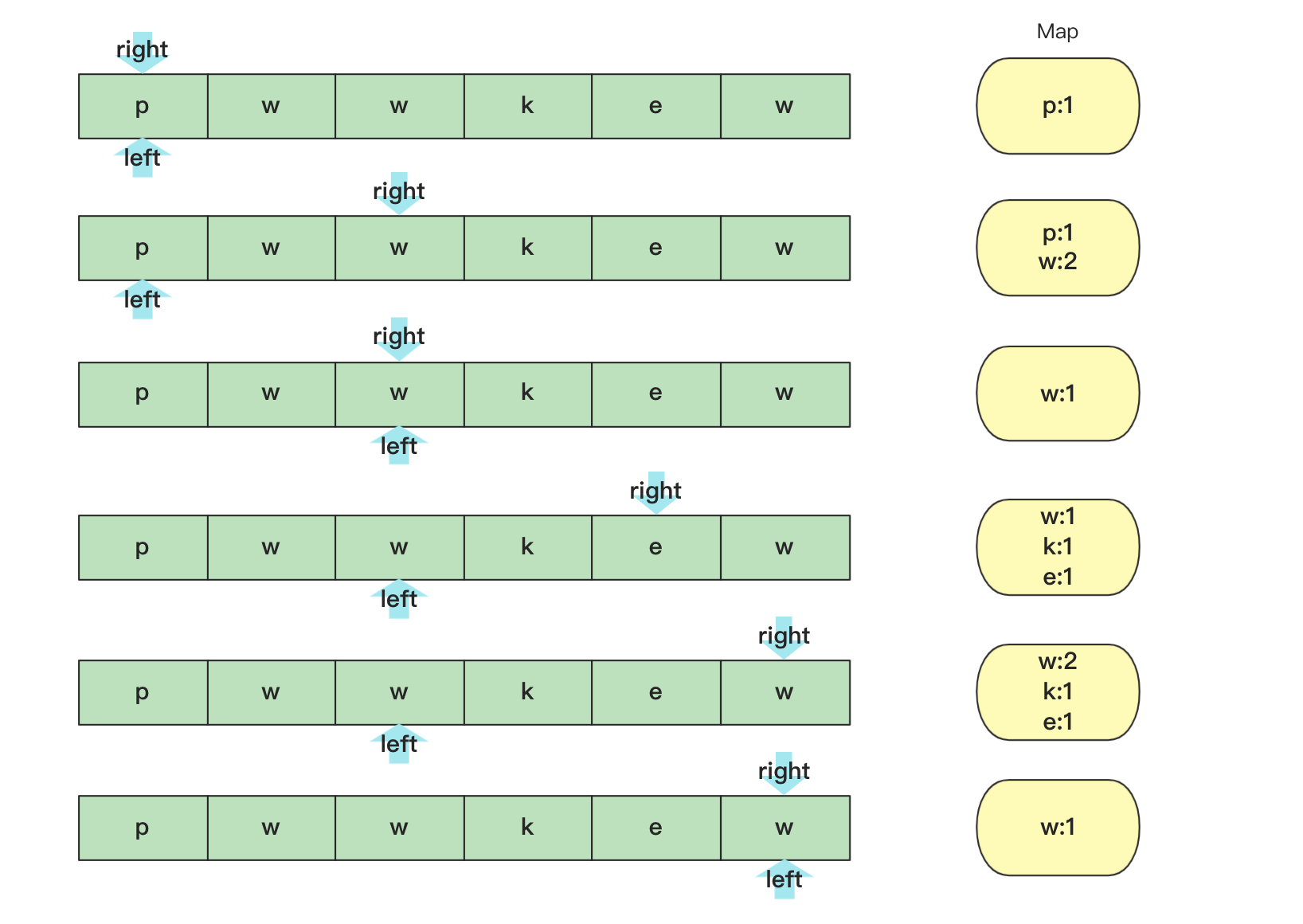class Solution {
public int lengthOfLongestSubstring(String s) {
int a[]=new int;
int max=0;//记录最大
int l=0;//left 用i 当成right，当有重复左就往右
for(int i=0;i<s.length();i++)
{
a[s.charAt(i)]++;
while (a[s.charAt(i)]>1) {
a[s.charAt(l++)]--;
}
if(i-l+1>max)
max=i-l+1;
}
return max;
}
}


#### 0X10 排序public void quicksort(int [] a,int left,int right)
{
int low=left;
int high=right;
//下面两句的顺序一定不能混，否则会产生数组越界！！！very important！！！
if(low>high)//作为判断是否截止条件
return;
int k=a[low];//额外空间k，取最左侧的一个作为衡量，最后要求左侧都比它小，右侧都比它大。
while(low<high)//这一轮要求把左侧小于a[low],右侧大于a[low]。
{
while(low<high&&a[high]>=k)//右侧找到第一个小于k的停止
{
high--;
}
//这样就找到第一个比它小的了
a[low]=a[high];//放到low位置
while(low<high&&a[low]<=k)//在low往右找到第一个大于k的，放到右侧a[high]位置
{
low++;
}
a[high]=a[low];
}
a[low]=k;//赋值然后左右递归分治求之
quicksort(a, left, low-1);
quicksort(a, low+1, right);
}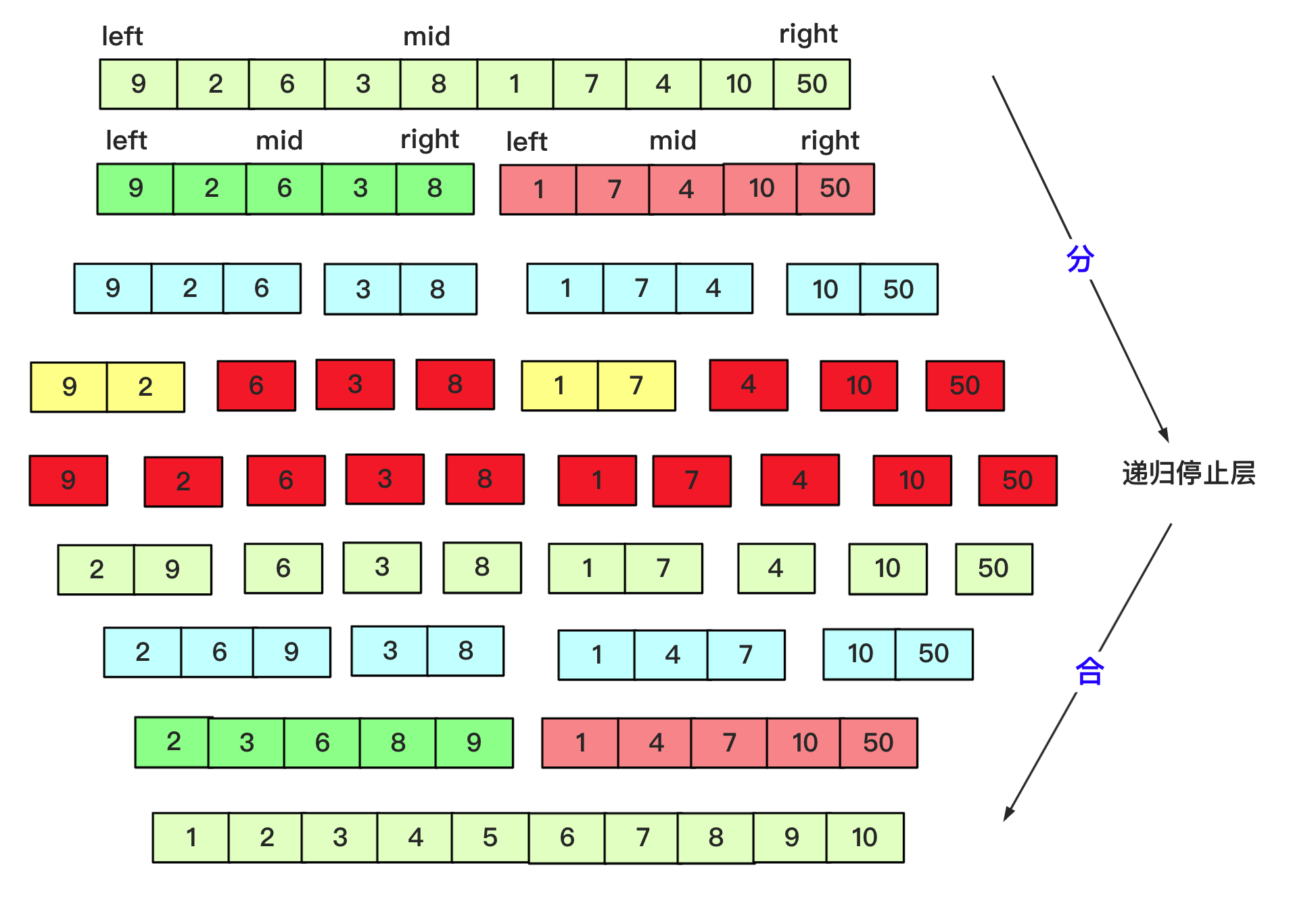private static void mergesort(int[] array, int left, int right) {
int mid=(left+right)/2;
if(left<right)
{
mergesort(array, left, mid);
mergesort(array, mid+1, right);
merge(array, left,mid, right);
}
}

private static void merge(int[] array, int l, int mid, int r) {
int lindex=l;int rindex=mid+1;
int team[]=new int[r-l+1];
int teamindex=0;
while (lindex<=mid&&rindex<=r) {//先左右比较合并
if(array[lindex]<=array[rindex])
{
team[teamindex++]=array[lindex++];
}
else {
team[teamindex++]=array[rindex++];
}
}
while(lindex<=mid)//当一个越界后剩余按序列添加即可
{
team[teamindex++]=array[lindex++];

}
while(rindex<=r)
{
team[teamindex++]=array[rindex++];
}
for(int i=0;i<teamindex;i++)
{
array[l+i]=team[i];
}
}


#### 结语https://item.jd.com/13014179.html

http://product.dangdang.com/29334623.html

09-17896
08-231万+
10-13
03-161万+
12-031267
10-21851
02-102364
02-091658
04-162373
03-036827
10-294731
12-241646
10-31
05-17
01-17580
08-04
06-15
03-06

### “相关推荐”对你有帮助么？

•非常没帮助
•没帮助
•一般
•有帮助
•非常有帮助被折叠的  条评论 为什么被折叠?到【灌水乐园】发言Big sai

¥1 ¥2 ¥4 ¥6 ¥10 ¥20获取中扫码支付点击重新获取扫码支付1.余额是钱包充值的虚拟货币，按照1:1的比例进行支付金额的抵扣。
2.余额无法直接购买下载，可以购买VIP、付费专栏及课程。余额充值